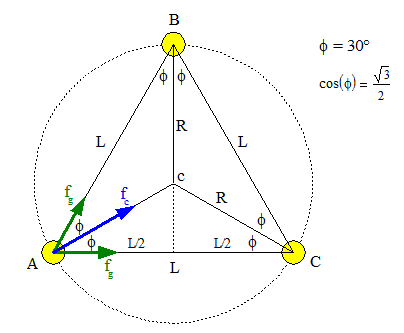# Question on Kepler's Law

• i_hate_math

## Homework Statement

Three identical stars of mass M = 8.9 x 1030 kg form an equilateral triangle that rotates around the triangle's center as the stars move in a common circle about that center. The triangle has edge length L = 2.8 x 1010 m. What is the speed of the stars?

## Homework Equations

Kepler's 3rd Law: T^2=(4*π^2/GM)*R^3
v=2πR/T

## The Attempt at a Solution

I used L to find an expression for R, namely R=L/sqrt(3), since its a equilateral triangle and L one of the sides (this is probably where I went wrong). I then used the two equations above to calculate the speed.
Now I know that the correct solution to this question is v=(GM/L)^0.5=1.456*10^5,
can someone pls explain to me why it is so?

Start with the basics: centripetal force being provided by the net gravitational force on any given star in the system.•i_hate_math
Start with the basics: centripetal force being provided by the net gravitational force on any given star in the system.
View attachment 99491
I had m*v^2/R = G*m*3M/R^2, this led me to
v=sqrt(3GM/R)
and L=sqrt(3)R, could u tell me where I went wrong?

G*m*3M/R^2
That is the magnitude of the force exerted by (what exactly) in what direction?

That is the magnitude of the force exerted by (what exactly) in what direction?
I think this is the force exerted by the three suns and that is towards the centre of the circular orbit?

I think this is the force exerted by the three suns and that is towards the centre of the circular orbit?
Each sun is only pulled by two others. But anyway, it looks to me like you calculated the force exerted by a single sun towards itself (GMm/L2).